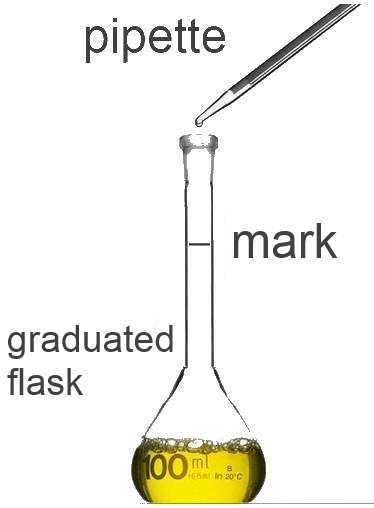MathJax.Hub.Config({ tex2jax: {inlineMath: [['$','$'], ['\$','\$']]} }); MathJax.Hub.Config({ "HTML-CSS": { linebreaks: { automatic: true } }, SVG: { linebreaks: { automatic: true } } });Search:

# Dilutions

## VocabularyA concentrated solution is a solution with high concentration. A diluted solution is a solution with low concentration. Using a pipette a well defined volume of the concentrated solution is introduced in a graduated flask. The solvent is then introduced up to the mark, the flask is shaken. Finally we obtain a well defined volume of a diluted solution.

## Principle

In the case of aqueous solutions (where the solvant is water), volumes may be added together:

V(diluted solution) = V(concentrated solution) + V(added water)

During dilution the number of moles of solute did not change:

n(solute in the diluted solution) = n(solute in the concentrated solution)